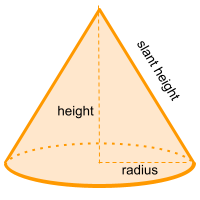Home » Non-polyhedra » Cone

# Volume of a ConeVolume =

## Volume calculator for a cone

Enter the radius and height of the cone to get the volume automatically

## Description, how many faces, edges and vertices are there in a cone

The cone can be generated by rotating a triangle on its own axis generating a three-dimensional shape, the cone. This solid shape has two faces, a circle as the base and a curved face, has one vertex at the other end of the base (the tip of the cone) and one edge.

When the cone has its vertex right in the middle of the base, then it is called a regular cone, in the case that the vertex is aligned to another side that is not the center, it is called an oblique cone.

## Examples of a cone

We can find many objects that look like a cone, examples of cone shaped objects are: birthday hat, ice-cream cone or traffic cone. Can you think of any other examples? leave a comment in the comment section at the bottom of the page.

## Formula for the volume of a cone

The same formula to calculate volume is used for both the regular cone and the oblique cone. You need π (Pi = ~ 3.14) over 3, then multiply by the radius to the power of two and finally multiply by the height.

If you don't know the height or the radius, you can use the slant height to obtain some of the other data, this using the Pythagorean theorem. You can also use the online calculator to get the volume of a cone automatically .

Volume =